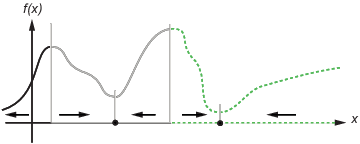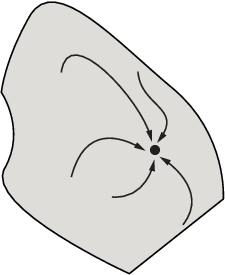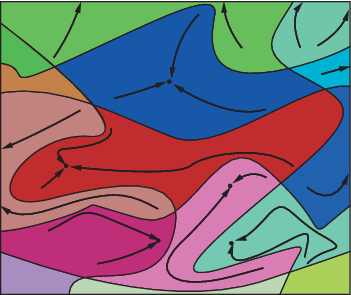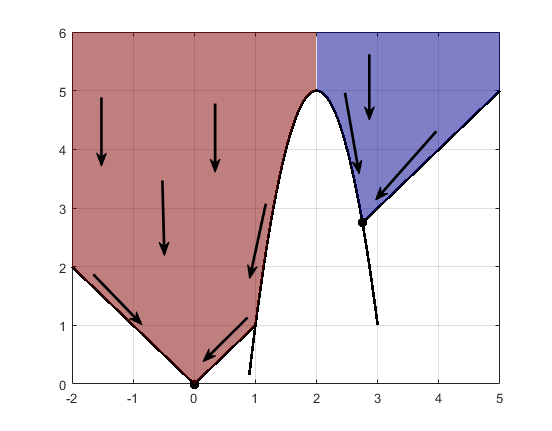• 最陡下降方程，即ddtx(t)=−∇f(x(t)),会生成一条路径 x(t)，该路径随着 t 的增长而抵达局部最小值。通常，彼此靠近的初始值 x(0) 给出趋向于相同最小值点的最陡下降路径。最陡下降的吸引盆是导向相同局部最小值的一...

吸引盆

如果目标函数 f(x) 是平滑的，则向量 –∇f(x) 指向 f(x) 下降最快的方向。最陡下降方程，即

ddtx(t)=−∇f(x(t)),

会生成一条路径 x(t)，该路径随着 t 的增长而抵达局部最小值。通常，彼此靠近的初始值 x(0) 给出趋向于相同最小值点的最陡下降路径。最陡下降的吸引盆是导向相同局部最小值的一组初始值。

下图显示两个一维最小值。该图使用不同线型显示不同的吸引盆，并用箭头指示最陡下降的方向。对于此图以及后面的图，黑点表示局部最小值。从 x(0) 点开始，每条最陡下降路径都抵达包含 x(0) 的盆中的黑点。

一维盆下图显示最陡下降路径在更多维度的情形中变得更为复杂。

显示来自不同起点的最陡下降路径的吸引盆下图显示更加复杂的路径和吸引盆。

多个吸引盆约束可以将一个吸引盆分成几个部分。例如，假设在以下约束条件下求 y 的最小值：

y ≥ |x|

y ≥ 5 –

4(x–2)2。

下图显示两个具有最终点的吸引盆。myabs = @(x) abs(x);

mycurve = @(x) 5 - 4.*(x-2).^2;

myline = @(x) max(myabs(x),mycurve(x));

mybrdr = @(x) 1 + heaviside(x-2);

myarea = @(x,y) heaviside(y - myline(x)).*mybrdr(x);

% myarea = 0 where infeasible, 1 when x < 2, 2 when x > 2

marinv = @(x,y) 1./myarea(x,y); % removes the infeasible region

plot(0,0,'k.','MarkerSize',25,'LineWidth',2);

hold on

grid on

plot(11/4,11/4,'k.','MarkerSize',25,'LineWidth',2);

fplot(mycurve,'k',[.9 3],'LineWidth',2)

fplot(myline,[-2 5],'k','LineWidth',2)

fsurf(marinv,[-2 5 -1,6],'EdgeColor','none','FaceAlpha',0.5)

colormap jet

在命令行中输入 plottools 以使用箭头绘制工具添加箭头。

最陡下降路径是向下一直延伸到约束边界的直线。从约束边界开始，最陡下降路径沿边界向下行进。最终点是 (0,0) 或 (11/4,11/4)，具体取决于初始 x 值是高于还是低于 2。

展开全文• matlab局部最优和全局最优算法

万次阅读 多人点赞 2016-03-03 14:06:10
在实际的工作和生活过程中...优化问题一般分为局部最优全局最优局部最优，就是在函数值空间的一个有限区域内寻找最小值；而全局最优，是在函数值空间整个区域寻找最小值问题。 函数局部最小点是那种它的函数值

转自http://blog.sciencenet.cn/blog-922140-850587.html

在实际的工作和生活过程中，优化问题无处不在，比如资源如何分配效益最高，拟合问题，最小最大值问题等等。优化问题一般分为局部最优和全局最优，局部最优，就是在函数值空间的一个有限区域内寻找最小值；而全局最优，是在函数值空间整个区域寻找最小值问题。

• 函数局部最小点是那种它的函数值小于或等于附近点的点。但是有可能大于较远距离的点。

• 全局最小点是那种它的函数值小于或等于所有的可行点。

matlab中的提供的传统优化工具箱（Optimization Tool），能实现局部最优，但要得全局最优，则要用全局最优化算法（Global  Optimization Tool ），主要包括：
1. GlobalSearch） 全局搜索和（MultiStart）多起点方法产生若干起始点，然后它们用局部求解器去找到起始点吸引盆处的最优点。

2. ga  遗传算法用一组起始点（称为种群），通过迭代从种群中产生更好的点，只要初始种群覆盖几个盆，GA就能检查几个盆。

3. simulannealbnd）模拟退火完成一个随机搜索，通常，模拟退火算法接受一个点，只要这个点比前面那个好，它也偶而接受一个比较糟的点，目的是转向不同的盆。

4. patternsearch ）模式搜索算法在接受一个点之前要看看其附近的一组点。假如附近的某些点属于不同的盆，模式搜索算法本质上时同时搜索若干个盆

下面我就一些具体例子，来说明各种优化方法：
（1）先看一个求最小值的普通优化问题
 1 2 3 4 5 6 7 8 9 10 11 12 13 14 15 16 17 18 19 20 21 22 %%目标函数 f = @(x) x.*sin(x) + x.*cos(2.*x); %% 的取值范围 lb = 0; ub = 10; %% 寻找最小值和绘图 x0 = [0 1 3 6 8 10]; hf = figure; for i=1:6    x(i) = fmincon(f,x0(i),[],[],[],[],lb,ub,[],...                   optimset('Algorithm','SQP','Disp','none'));    subplot(2,3,i)    ezplot(f,[lb ub]);    hold on    plot(x0(i),f(x0(i)),'k+')    plot(x(i),f(x(i)),'ro')    hold off    title(['Starting at ',num2str(x0(i))])    if i == 1 || i == 4        ylabel('x sin(x) + x cos(2 x)')    end end可以看出，初值x0不同，得到的结果截然不同，这说明这种求解器，能寻找局部最优，但不一定是全局最优，在起点为8时，取得全局最优。

我们换一种求解器：fminbound,这种求解器不需要给点初值。

 1 2 3 4 5 6 7 8 x2 = fminbnd(f,lb,ub); figure ezplot(f,[lb ub]); hold on plot(x2,f(x2),'ro') hold off ylabel('x sin(x) + x cos(2 x)') title({'Solution using fminbnd.','Required no starting point!'})现在我们尝试全局最优的方法：GlobalSearch

 1 2 3 4 5 6 7 8 9 10 11 12 13 14 % Leason Learned: Use the appropriate solver for your problem type! %% But what if |fmincon| was the only choice? % Use globalSearch or MultiStart problem = createOptimProblem('fmincon','objective',f,'x0',x0(1),'lb',lb,...             'ub',ub,'options',optimset('Algorithm','SQP','Disp','none')); gs = GlobalSearch; xgs = run(gs,problem); figure ezplot(f,[lb ub]); hold on plot(xgs,f(xgs),'ro') hold off ylabel('x sin(x) + x cos(2 x)') title('Solution using globalSearch.')因此全局最优的方法能够获取全局最优。

（2）再看一个线性拟合的问题:
 1 2 3 4 5 6 7 8 9 10 11 12 13 14 15 16 17 18 19 20 21 22 close all, clear all, clc %% Pharmacokinetic Data t = [ 3.92,  7.93, 11.89, 23.90, 47.87, 71.91, 93.85, 117.84 ]              %#ok<*NOPTS> c = [0.163, 0.679, 0.679, 0.388, 0.183, 0.125, 0.086, 0.0624 ]   plot(t,c,'o'), xlabel('t'), ylabel('c')   %% 3 Compartment Model model = @(b,t) b(1)*exp(-b(4)*t) + b(2)*exp(-b(5)*t) + b(3)*exp(-b(6)*t)   %% Define Optimization Problem   problem = createOptimProblem('lsqcurvefit', ...                             'objective', model, ...                             'xdata', t, 'ydata', c, ...                             'x0',ones(1,6),...                             'lb', [-10 -10 -10  0   0   0 ],...                             'ub', [ 10  10  10 0.5 0.5 0.5], ...                             'options',optimset('OutputFcn',...                             @curvefittingPlotIterates)) %% solve b = lsqcurvefit(problem)

结果：最小二乘拟合结果误差较大现在我们尝试全局最优方法：MultiStart  1 2 3 4 5 6 7 8 9 10 %% Multistart ms = MultiStart                                                             [b,fval,exitflag,output,solutions] = run(ms, problem, 50)                   %#ok<*NASGU,*ASGLU>   %% curvefittingPlotIterates(solutions)   %% problem.options.OutputFcn = {}; tic, [b,fval,exitflag,output,solutions] = run(ms, problem, 100), toc  %计算算法的时间可以看出全局优化结果较好，误差较小。
这种算法的运行时间 ：Elapsed time is 6.139324 seconds.

现在我使用并行计算的方式解决：
 1 2 3 4 5 %% Parallel Version matlabpool open 2 %开启两个matlab并行计算 ms.UseParallel = 'always' %开启并行计算 tic, [bp,fvalp,exitflagp,outputp,solutionsp] = run(ms, problem, 100); toc matlabpool close
结果：14 out of 100 local solver runs converged with a positive local solver exit flag.
Elapsed time is 4.358762 seconds.
Sending a stop signal to all the labs ... stopped.
可以看出，运行时间减少，提高了效率。

（3）再看一个寻找最小值的问题
 1 2 3 4 5 6 7 8 9 10 11 12 13 14 15 16 17 18 19 20 21 22 23 %% Objective Function % We wish find the minimum of the |peaks| function clear all, close all, clc peaks   %% Nonlinear Constraint Function % Subject to a nonlinear constraint defined by a circular region of radius % three around the origin type circularConstraint   %% Define Optimization Problem problem = createOptimProblem('fmincon',...                             'objective',@(x) peaks(x(1),x(2)), ...                             'nonlcon',@circularConstraint,...                             'x0',[-1 -1],...                             'lb',[-3 -3],...                             'ub',[3 3],...                             'options',optimset('OutputFcn',...                                                @peaksPlotIterates))                               %% Run the solver |fmincon| from the inital point % We can see the solution is not the global minimum [x,f] = fmincon(problem)这种方法只能寻找局部最优。
现在用全局优化算法：
 1 2 3 4 5 6 7 %% Use |MultiStart| to Find the Global Minimum % Define the multistart solver close all ms = MultiStart %这里可以换成GlobalSearch %% Run |Multistart| % Well use 5 starting points [x,f,exitflag,output,solutions] = run(ms, problem, 5)（4）再举一个模拟退火即模式搜索的算法 ：
[x fval] = simulannealbnd(@objfun,x0,lb,ub,options)

 1 2 3 4 5 6 7 8 9 10 11 12 13 14 15 16 17 18 19 20 21 22 23 24 25 26 27 28 29 30 31 32 33 34 35 36 37 %% Objective Function % We wish find the minimum of the |peaks| function clear all, close all, clc peaks   %% Nonlinear Constraint Function % Subject to a nonlinear constraint defined by a circular region of radius % three around the origin type circularConstraint   %% Define Optimization Problem problem = createOptimProblem('fmincon',...                             'objective',@(x) peaks(x(1),x(2)), ...                             'nonlcon',@circularConstraint,...                             'x0',[-1 -1],...                             'lb',[-3 -3],...                             'ub',[3 3],...                             'options',optimset('OutputFcn',...                                                @peaksPlotIterates))                               %% Run the solver |fmincon| from the inital point % We can see the solution is not the global minimum [x,f] = fmincon(problem)                                                       %% Use Simmulated Annealing to Find the Global Minimum % Solve the problem using simmulated annealing.  Note that simmulated % annealing does not support nonlinear so we need to account for this in % the objective function. problem.solver  = 'simulannealbnd'; problem.objective = @(x) peaks(x(1),x(2)) + (x(1)^2 + x(2)^2 - 9); problem.options = saoptimset('OutputFcn',@peaksPlotIterates,...                             'Display','iter',...                             'InitialTemperature',10,...                             'MaxIter',300)   [x,f] = simulannealbnd(problem) f = peaks(x(1),x(2))Use Pattern Search to Find the Global Minimum
 1 2 3 4 5 6 7 8 %% Use Pattern Search to Find the Global Minimum % Solve the problem using pattern search. problem.solver  = 'patternsearch'; problem.options = psoptimset('OutputFcn',@peaksPlotIterates,...                             'Display','iter',...                             'SearchMethod',{@searchlhs})   [x,f] = patternsearch(problem)

展开全文matlab 算法 优化
• 贪心题目：从局部最优解得到全局最优解。 贪心策略如下：先对数据按照金额从小到大进行排序。对于金额大于C的纸币，直接全部取出；之后进行若干次循环，每次循环中先从大到小尽可能取到小于C的最大金额，之后再...

一、题目

Description

As a reward for record milk production, Farmer John has decided to start paying Bessie the cow a small weekly allowance. FJ has a set of coins in N (1 <= N <= 20) different denominations, where each denomination of coin evenly divides the next-larger denomination (e.g., 1 cent coins, 5 cent coins, 10 cent coins, and 50 cent coins).Using the given set of coins, he would like to pay Bessie at least some given amount of money C (1 <= C <= 100,000,000) every week.Please help him ompute the maximum number of weeks he can pay Bessie.

Input

• Line 1: Two space-separated integers: N and C

• Lines 2..N+1: Each line corresponds to a denomination of coin and contains two integers: the value V (1 <= V <= 100,000,000) of the denomination, and the number of coins B (1 <= B <= 1,000,000) of this denomation in Farmer John's possession.
Output

• Line 1: A single integer that is the number of weeks Farmer John can pay Bessie at least C allowance

3 6
10 1
1 100
5 120

111

Hint

INPUT DETAILS:
FJ would like to pay Bessie 6 cents per week. He has 100 1-cent coins,120 5-cent coins, and 1 10-cent coin.

OUTPUT DETAILS:
FJ can overpay Bessie with the one 10-cent coin for 1 week, then pay Bessie two 5-cent coins for 10 weeks and then pay Bessie one 1-cent coin and one 5-cent coin for 100 weeks.

二、思路&心得

• 贪心题目：从局部最优解得到全局最优解。
• 贪心策略如下：先对数据按照金额从小到大进行排序。对于金额大于C的纸币，直接全部取出；之后进行若干次循环，每次循环中先从大到小尽可能取到小于C的最大金额，之后再从小到大尽可能凑满C，允许超出一个当前最小金额值，一次处理结束后更新相应金额的数量。
• 这个贪心策略的数学化证明暂时没有想到，题目中还给出“金额之间还有确定的倍数关系”，也不清楚这个信息在算法中具体体现了什么作用。对于这个贪心策略，一个较为直观的解释如下：类比生活中买东西，当消费了一定金额后，我们肯定都是使用尽可能多的大面值的RMB，再使用小面值的，整个题目的思想应该跟这个差不多，我们生活中都下意识使用了很多的贪心策略。

• PS：做这个题目时，实在被坑了好久，花了三四个小时，RE + WA无数次才过，主要的问题还是自己一开始上手时，所使用的贪心策略完全错误，尝试了多种，并且举反例证明之后才逐渐找到了正确的贪心策略。

三、代码

#include<cstdio>
#include<climits>
#include<algorithm>
using namespace std;
const int MAX_N = 25;

int N, C;

int ans;

int use[MAX_N];

struct Money {
int value;
int number;
} a[MAX_N];

bool cmp(Money a, Money b) {
return a.value < b.value;
}

void solve() {
int i, start = -1;
int min_num, dist;
//计算所有面额大于C的数据
for (i = N - 1; i >= 0; i --) {
if (a[i].value >= C) {
ans += a[i].number;
} else {
start = i;
break;
}
}
while (1) {
fill(use, use + N, 0);
dist = C;
//从大到小取到小于C的最大数值
for (i = start; i >= 0; i --) {
if (a[i].number) {
min_num = min(dist / a[i].value, a[i].number);
use[i] = min_num;
dist -= a[i].value * min_num;
}
}
//从小到大凑满C
if (dist > 0) {
for (int i = 0; i <= start; i ++) {
if (a[i].number) {
min_num = min((dist + a[i].value - 1) / a[i].value, a[i].number - use[i]);
use[i] += min_num;
dist -= a[i].value * min_num;
if (dist <= 0) break;
}
}
}
if (dist > 0) break;
//数量更新
min_num = INT_MAX;
for (i = 0; i <= start; i ++) {
if (use[i])
min_num = min(min_num, a[i].number / use[i]);
}
for (i = 0; i <= start; i ++) {
if (use[i]) {
a[i].number -= min_num * use[i];
}
}
ans += min_num;
}
printf("%d\n", ans);
}

int main() {
while (~scanf("%d %d", &N, &C)) {
ans = 0;
for (int i = 0; i < N; i ++) {
scanf("%d %d", &a[i].value, &a[i].number);
}
sort(a, a + N, cmp);
solve();
}
return 0;
}

转载于:https://www.cnblogs.com/CSLaker/p/7307771.html

展开全文• 全局最优和局部最优的理解

万次阅读 2019-09-28 08:52:47
2、自己想的，如果是凸函数，或者是凸规划，那么只有一个局部最优解，这个局部最优解 就是 全局最优解。 我们在求解的时候，思路上都是找一个局部最优解，或者说是通过迭代运算，找目标函数值下降的解，直到两个解...
• 198. House Robber You are a professional robber planning to rob houses along a street. Each house has a certain amount of money stashed, the only constraint stopping you from robbing each of them动态规划
• 该算法在粒子更新速度的过程中, 将前几轮粒子搜索的历史全局最优信息本轮局部最优粒子信息结合,增加粒子搜索信息的多样性。另外,根据2种信息的结合方式不同,将基本算法扩展成3种扩展型算法。6个典型函数的仿真实验...
• 这是一道非常经典的动态规划的题目，用到的思路我们在别的动态规划题目中也很常用，以后我们称为”局部最优全局最优解法“。 基本思路是这样的，在每一步，我们维护两个变量，一个是全局最优，就是到当前元素为止...
• 文章目录基本知识回顾如何证明问题具有贪心选择性质样例一：活动安排问题问题描述总有一个以贪心选择为开始的最优解样例二、最优装载的贪心性证明问题描述证明过程分析总结 基本知识回顾 贪心算法的基本要素 最...贪心算法
• 我以前主要搞优化算法，但对于大多数数值最优化算法求的都是局部最优，当然也些能求全局最优，就是凸集上的凸问题，简单说这类问题就一个局部最优解，当然也就是全局最优了。多局部极值的问题的全局最优问题还没有...算法 优化
• 深度神经网络“容易收敛到局部最优”，很可能是一种想象，实际情况是，我们可能从来没有找到过“局部最优”，更别说全局最优了。 很多人都有一种看法，就是“局部最优是神经网络优化的主要难点”。这来源于一维优化...
• 局部最优和鞍点   造成神经网络难以优化的一个重要（乃至主要）原因不是高维优化问题中有很多局部极值，而是存在大量鞍点。   吴恩达视频中讲的，虽然没有理论的证明，局部最小值就是全局最小值，但是很多实际的...
• 局部最优与鞍点问题

千次阅读 2020-06-26 12:05:53
梯度下降法或者某个算法可能困在一个局部最优中，而不会抵达全局最优。但是，问题的关键在于，低维特征（图示两维）让我们对局部最优产生误解。 事实上，如果你要创建一个神经网络，通常梯度为零的点并不是这个图中...
• 因此，MORELA使得发现数学函数的全局最优成为可能，因为它是在前一个学习情节中使用子环境寻求的最佳解决方案的基础上寻求的。 已使用从文献中描述的其他优化方法获得的结果测试了MORELA的性能。 结果表明，就采用...
• Matlab全局优化与局部优化

千次阅读 2018-04-01 10:15:51
优化问题一般分为局部最优全局最优局部最优，就是在函数值空间的一个有限区域内寻找最小值；而全局最优，是在函数值空间整个区域寻找最小值问题。函数局部最小点是那种它的函数值小于或等于附近点的点。但是有...
• 算法 - 局部最优的避免

万次阅读 2019-09-16 10:25:51
一般的启发式算法非常容易产生局部最优，或者说根本无法查证产生的最优解是否是全局的。这是因为对于大型系统或复杂的问题，一般的算法都着眼于从局部展开求解，以减少计算量和算法复杂度1。 通常我们希望得到的是...局部最优 避免局部最优
• 就是一句话，局部最优推出全局最优，找不出反例，试试贪心贪心算法 数据结构
• 这次我们依然使用“局部最优全局最优法”，不过这次维护的全局最优要分成两个变量：step最优和step-1步最优（假设当前步数是step步），如果我们的坐标  i  走到了超过step-1步最远达到的位置lastReach，也就是说...Array Greedy leetcode
• 深度学习中局部最优问题

万次阅读 2019-02-01 22:49:33
局部最优的问题(The problem of local optima) ...梯度下降法或者某个算法可能困在一个局部最优中，而不会抵达全局最优。但是，问题的关键在于，低维特征（图示两维）让我们对局部最优产生误解。 事实上，...
• 局部最优的问题(The problem of local optima) 在深度学习研究早期，人们总是担心优化算法会困在极差的局部最优，不过随着深度学习理论不断发展，我们对局部最优的理解也发生了改变。我向你展示一下现在我们怎么看待...
• 乘积最大子序列 java （局部最优全局最优解法） 给定一个整数数组 nums ，找到一个具有最大和的连续子数组（子数组最少包含一个元素），返回其最大和。 示例: 输入: [-2,1,-3,4,-1,2,1,-5,4], 输出: 6 解释: ...
• 动态规划求解，我们维护两个变量，一个局部最优，一个全局最优。一个表示到目前为止我们能达到的最远距离，即local = A[i]+i, 另外一个是当前一步出发能跳到的最远距离，global = max(global, local). 确定了递推...Array Greedy leetcode
• 关于局部最优

2019-12-12 16:09:17
在开始学习梯度下降的时候，总会有这样的疑问：梯度下降只能到达局部最优，万一到达了一个较大的局部最优，错过了较小的全局最优或是另外一个更小的局部最优，那么是不是算法是失败呢？ 其实在机器学习的大数据背景...
• 基于全局最优-局部最优粒子群算法的PID控制.pdf
• 1.概念： 贪心算法（又称贪婪算法）是指，在对问题求解时，总是做出在当前看来是最好的选择。也就是说，不从整体最优上加以考虑，算法得到...3.存在局部最优问题的体现： 找零钱时，如果零钱面额是【1，5，10】，仿佛没贪心算法
• 如何跳出局部最优

千次阅读 2019-07-24 22:24:27
随机梯度下降，加入随机因素，每次取一个样本计算梯度，因为单点的最优方向可能不是全局的最优方向，表现在图像上就是在寻找全局最优的路上饶了很多弯路才到达最优点。 使用模拟退火算法，每次以一定的概率允许移动...
• 也就是说贪心算法并不从整体最优考虑，它所作出的选择只是在某种意义上的局部最优选择。当然，希望贪心算法得到的最终结果也是整体最优的。虽然贪心算法不能对所有问题都得到整体最优解，但对许多问题它能产生整体最...贪心算法
• 除了因为局部最优在高维中出现的概率小，我们不必为它太过烦恼(一定要注意是在高维情况下)，还有就是局部最优本来也并非想象中那么可怕，我们担心的是找到的解和全局最优相差很大，因为最重要的是要找到相对较低的...鞍点 局部最优...